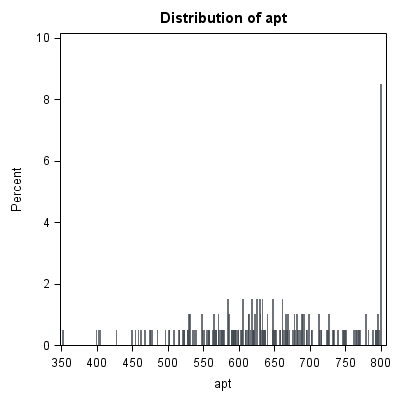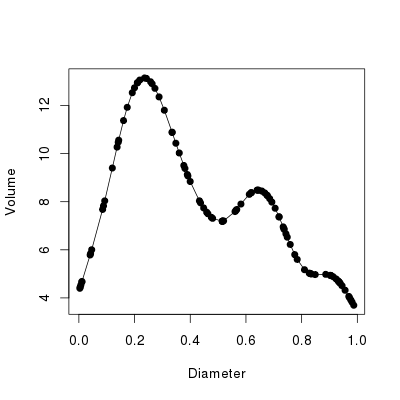# Difference between Categorical and Numerical Data

Considering the advancements made in the field of technical sciences, it has become possible to develop complicated mathematical and statistical data using a range of applications such as the environment, energy, chemical sciences, biology, healthcare, politics, law and business statistics. More and more companies are now showing interest in building up data that can help improve control, application and management. However, the main focus these days is on extracting meaningful information by looking into the knowledge provided by the information – the data.

Based on common classification and taxonomy theory, data variables are usually categorised as either numerical or categorical. Numerical category represents the collection of quantitative data whereas the categorical data set of variables represents qualitative information.

In numerical sciences, a collection of data items is known as a data set. A collection of statistical data is used to represent an entity and its performance. A mix of variables is required to explain the characteristics of a data item. For examples, the rate of inflation for a country can be calculated by using constant values and variables.  Variables and constants will represent various aspects of that country’s performance.

When two or more variables are added to the mix, the data set is known as the multivariate data set. The items in a data set can be considered as some points in a multidimensional space. Each dimension will then represent a variable. By calculating the variables, it will be possible to compute the performance or inflation in the case of a country. The m rows  x n columns matrices technique is typically used to devise a standard format for structuring multidimensional and multivariate data.

Categorical data belongs to the nominal type, whereas numerical data almost always has connections with internal, ratio or ordinal type. Methods used to assess or evaluate numerical data include statistical techniques and time series in descriptive statistics. Categorical data is evaluated using very different methods with entirely different applications. Graphical and descriptive methods are commonly employed for categorical data in addition to performing non-parametric tests.

### Instructions

• 1

Categorical

Categorical data of variables therefore can be a collection of different names or a numerical technique to determine the characteristics by putting the items in either ascending or descending order. It is vital to classify data sets as categorical where different visual and analytic systems are applied. With categorical data it is not possible to measure distance against the numerical distance and appropriate visual and analytic techniques must be applied.

Image courtesy: ats.ucla.edu• 2

Numerical

Numerical data is usually based on the similarity between items or a numerical difference. It is easy to collect numerical data and use it as a performance indicator.

Image courtesy: r-bloggers.com•